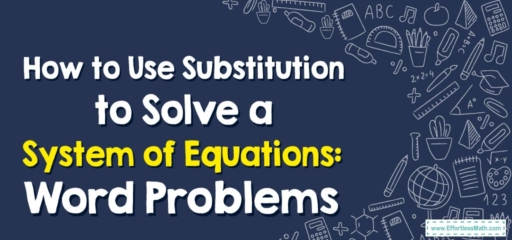# How to Use Substitution to Solve a System of Equations: Word Problems

Solving systems of equations is a fundamental concept in algebra, and it plays a crucial role in various fields such as engineering, physics, and economics. One popular method to solve these systems is substitution, which involves substituting one variable with an expression from another equation. This article aims to provide a comprehensive guide on using substitution to solve a system of equations, particularly focusing on word problems.## A Step-by-step Guide to Using Substitution to Solve a System of Equations: Word Problems

Let’s go through a step-by-step guide on how to approach these problems.

### Step 1: Understand the Problem

Start by carefully reading the word problem. Identify the unknowns and what you need to solve for.

Example Problem: A movie theater sells adult tickets for $$10$$ and children tickets for $$6$$. If the theater sold $$100$$ tickets total and made $$800$$, how many adult tickets and children tickets were sold?

The unknowns here are the number of adult and children tickets sold, and we need to find these values.

### Step 2: Define the Variables

Next, define variables to represent the unknowns.

For our example:

• Let’s let ‘$$A$$’ represent the number of adult tickets sold.
• Let’s let ‘$$C$$’ represent the number of children’s tickets sold.

### Step 3: Formulate the Equations

Translate the information from the word problem into mathematical equations.

In our example, we can formulate two equations from the problem:

1. $$A + C = 100$$ (The total number of tickets sold is $$100$$)
2. $$10A + 6C = 800$$ (The total revenue from the tickets is $$800$$)

### Step 4: Solve One Equation for One Variable

The next step is to solve one of the equations for one variable. It’s usually easier to choose the equation and variable that will be the simplest to solve. In this case, we can solve the first equation for ‘$$A$$’:

$$A = 100 – C$$

### Step 5: Substitute the Expression into the Other Equation

Now that we have ‘$$A$$’ in terms of ‘$$C$$’, substitute this expression into the other equation (in place of ‘$$A$$’):

$$10(100 – C) + 6C = 800$$

$$1000 – 10C + 6C = 800$$

$$4C = 100$$

$$C = 50$$

### Step 6: Solve for the Other Variable

Substitute $$C= 50$$ into the first equation to solve for ‘$$A$$’:

$$A + 50 = 100$$

$$A = 50$$

Always check your answer to ensure it makes sense in the context of the problem. Substitute $$A= 50$$ and $$C= 50$$ into both original equations to verify that they are correct:

1. $$50 + 50 = 100$$ (This is correct)
2. $$10(50) + 6(50) = 800$$ (This is also correct)

Therefore, the theater sold $$50$$ adult tickets and $$50$$ children’s tickets.

This is a basic walkthrough of using substitution to solve a system of equations. Keep in mind that some problems may be more complex and require additional steps, but the fundamental process is the same.

### What people say about "How to Use Substitution to Solve a System of Equations: Word Problems - Effortless Math: We Help Students Learn to LOVE Mathematics"?

No one replied yet.

X
30% OFF

Limited time only!

Save Over 30%

SAVE $5 It was$16.99 now it is \$11.99